Early Evidence for Dark Matter: The Virial Theorem and Rotation Curves

Alex Sugarbaker December 2, 2007

(Submitted as coursework for Physics 210, Stanford University, Autumn 2007)

The ensuing discussion will explore some of the early evidence for dark matter, which is matter that cannot be observed directly via electromagnetic radiation. One of the earliest hints of the existence of dark matter was found by Fritz Zwicky in 1937 when he analyzed the dynamics of the Coma galaxy cluster . Further strong evidence was provided much later by Rubin, Ford, Thonnard and others in the form of galaxy rotation curves . Both of these methods show a discrepancy between the luminosity and the dynamics of large groups of stars. I will turn first to Zwicky's work, which relied upon the Virial Theorem.

The Virial Theorem

This section follows Zwicky's derivation . Consider a system of mutually interacting masses. Let ri be the position of mass mi with respect to the system center of mass. Furthermore, let Fi be the total force acting on this mass as a result of its interaction with all of the other masses in the system. Then, by Newton's second law,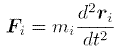Scalar multiplication of this equation by ri then yields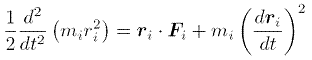Summing over all of the masses, time averaging, and letting vi be the velocity of the ith mass, we find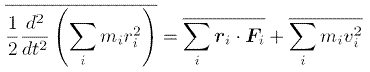If we further assume that the overall mass distribution (specifically ∑miri2) fluctuates about some equilibrium value, then the time average of the derivative on the left must be zero, leaving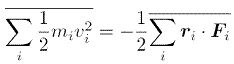This is the virial theorem, which relates a system's total kinetic energy (on the left) to its virial (the sum on the right)

Application of the Virial Theorem to the Coma Cluster

In what follows, I will show how Zwicky used the virial theorem to determine the approximate total mass of the Coma cluster of galaxies . This globular cluster consists of about 1000 galaxies distributed in a nearly spherically symmetric manner. Zwicky showed that the average galaxy mass determined using the virial theorem and the galaxy velocities (measured via redshift) differs significantly from the mass expected given the given the galaxy luminosities.

The virial for a system of masses interacting gravitationally can be simplified using Newton's third law, Fij = -Fji, where Fji is the force from mass j on mass i (and rji is the displacement from mass j to mass i). We get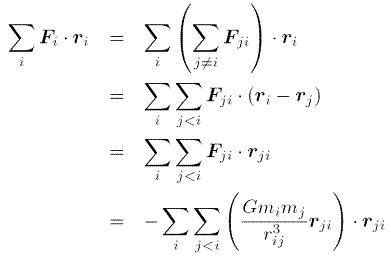Here, the third law has been used in the second step to reduce the number of terms in the sum by a factor of two and the final step has been achieved by explicitly writing the universal law of gravitation. Thus, for a system of gravitationally interacting masses, the virial theorem states that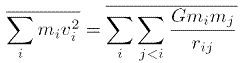The left side of this equation is just the total mass, M, of the galaxy cluster multiplied by the time and mass averaged squared velocity. The right side can be approximated to within constants of order unity as G*M2/R, where G is the gravitational constant and R is the radius of the galaxy cluster. (This can be obtained by assuming a uniform distribution of mass over a sphere of radius R. Zwicky discusses the applicability of this order of magnitude calculation even if the mass distribution varies significantly from uniformity.) To within constants of order unity, then, the total mass of the galaxy cluster is just the cluster radius times the mean squared velocity, divided by the gravitational constant.

Zwicky knew the radius of the Coma cluster to be 2*106 lightyears. The true galaxy velocities cannot be determined, but the line of sight velocities could be found via redshift. Using the mean squared line of sight velocity instead of the mean squared total velocity only introduces a factor of three (assuming spherical symmetry), so the result is not changed to within order of magnitude. Zwicky took 5*1015 cm2s-2 as the value for the time and mass averaged squared velocity. The total cluster mass is then about 7*1013 solar masses. Since the cluster contains about 1000 galaxies, this yields an average galactic mass of 7*1010 solar masses. However, the average galactic luminosity in the Coma cluster is found to be only 8.5*107 solar luminosities. Thus, the average mass to light ratio, in solar units, of the galaxies in the Coma cluster is approximately 800. This is several orders of magnitude greater than what one would expect if the mass of the Coma galaxies is mostly in stars (which should have mass to light ratios of order unity in solar units) . One plausible explanation for this discrepancy is dark matter, which contributes mass without increasing the galactic luminosity.

Zwicky even noted that a similar method could be applied to a single galaxy. However, in his time it was not possible to get accurate spectroscopic measurements from different parts of a given galaxy, so no determination of the mean squared velocity could be made. This discovery would await the improved spectroscopic techniques employed by Vera Rubin and her collaborators.

Galaxy Rotation Curves

Four decades after Zwicky's initial observations, Rubin, Ford, Thonnard, and others began to use new spectroscopic techniques to analyze the rotation curves of galaxies . These pivotal data provided the first pieces of evidence that large quantities of non-luminous mass might exist outside the visible region of most galaxies. While other explanations were originally suggested, these rotation curves are now commonly considered evidence for the presence of large quantities of dark matter.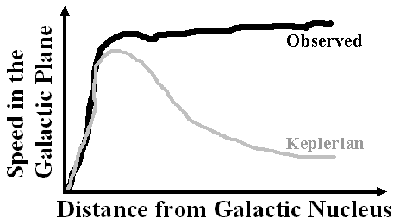Fig. 1: Approximation to a galactic rotation curve (black line). If most of the mass were very near the galactic center, then the velocities of the outermost stars would drop off in a Keplerian fashion (grey line).

The measurement of galactic rotation curves is fairly simple, provided that sufficiently accurate spectra may be obtained. The line of sight velocity of the luminous matter at various distances from the galactic center can be determined from the redshift of known spectral lines. The velocities in the galactic plane of spiral galaxies (the type of galaxy studied in ) can then be determined from the line of sight velocities using the visible angle of inclination of the galaxy. One of the major successes of  was the selection of high inclination galaxies for which the inclination angle can be determined most accurately. A plot of the speed in the galactic plane versus the distance from the galactic center is called the rotation curve for a galaxy (see Fig. 1).

Surprisingly, the rotation curves that were measured even for a wide range of galaxy sizes and luminosities exhibited a nearly flat (and even slightly increasing) region of velocities outside the galactic center. If most of the galactic mass were at the center of the galaxy (which is the source of most of the luminosity), then one would expect the velocities of the outermost stars to decrease with increasing distance. This velocity dependence would be like the Keplerian dependence observed in planetary motion. The velocity of the outermost stars orbiting with uniform circular motion can easily be determined by equating the gravitational force of attraction to the galactic core with the stellar mass times the centripetal acceleration. The velocity can then be seen to fall off as one over the square root of the orbital radius, contrary to the measured, nearly flat, rotation curves.

This provides further evidence for the possible existence of a large quantity of non-luminous galactic mass. Furthermore, these results suggest that much of this extra mass is farther from the galactic center than the luminous mass. While neither rotation curves nor Zwicky's early observations observations prove conclusively the existence of dark matter, they contributed to our early understanding of the subject and to the development of questions that we are still trying to answer.

© 2007 A.F. Sugarbaker. The author grants permission to copy, distribute and display this work in unaltered form, with attribution to the author, for noncommercial purposes only. All other rights, including commercial rights, are reserved to the author.

References

 F. Zwicky, Astrophys. J. 86, 217 (1937).

 V.C. Rubin, W.K. Ford, Jr. and N. Thonnard, Astrophys. J. 238, 471 (1980).

 H. Goldstein, C. Poole, and J. Safko, Classical Mechanics, 3rd Ed., (Addison-Wesley, 2002).